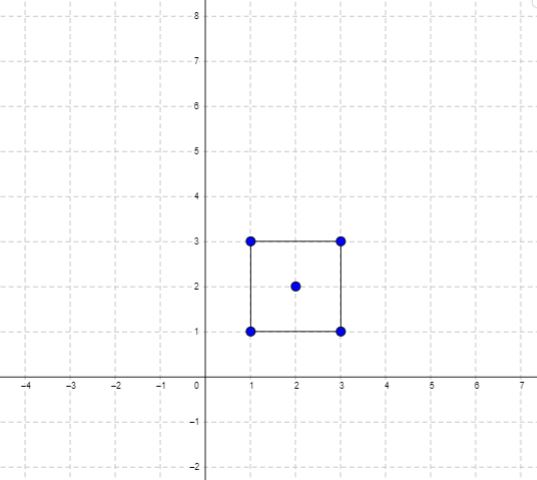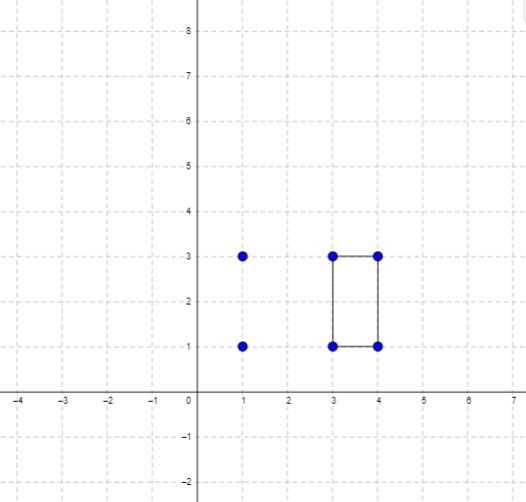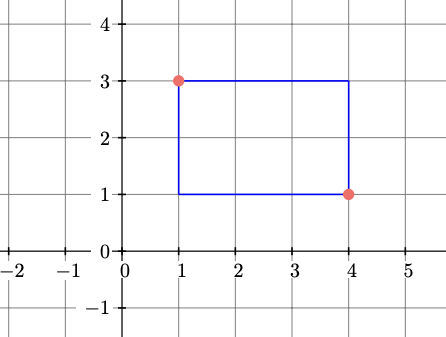# LeetCode 939. Minimum Area Rectangle Solution

You are given an array of points in the X-Y plane points where points[i] = [$x_i$, $y_i$].

Return the minimum area of a rectangle formed from these points, with sides parallel to the X and Y axes. If there is not any such rectangle, return 0.

Example 1:Input: points = [[1,1],[1,3],[3,1],[3,3],[2,2]]
Output: 4

Example 2:Input: points = [[1,1],[1,3],[3,1],[3,3],[4,1],[4,3]]
Output: 2

Constraints:

$1\leq$ points.length $\leq 500$
points[i].length == 2
$0 \leq x_i, y_i \leq 4 * 10^4$
All the given points are unique.

## Brute Force Solution

Since we need to form a rectangle from $4$ different points, we can check all combinations of $4$ points to see if it forms a rectangle. Then, we return the minimum area from a rectangle formed with these points. One key point is that we need to make sure the rectangle has positive area.

Let $N$ denote the size of points.

This algorithm runs in $\mathcal{O}(N^4)$.

## Full Solution

Let's try to optimize our algorithm to find all possible rectangles faster. One observation we can make is that a rectangle can be defined by two points that lie on one of the two diagonals.

### ExampleHere, the rectangle outlined in blue can be defined by the two red points at $(1,3)$ and $(4,1)$. It can also be defined by the two points $(1,1)$ and $(4,3)$ that lie on the other diagonal.

The two defining points have to be a part of points for the rectangle to exist. In addition, we need to check if the two other points in the rectangle exist in points. Specifically, let's denote the two defining points as $(x_1,y_1)$ and $(x_2,y_2)$. We'll need to check if $(x_1,y_2)$ and $(x_2,y_1)$ exist in points. This is where we can use a hashmap to do this operation in $\mathcal{O}(1)$. We'll also need to make sure the rectangle has positive area (i.e. $x_1 \neq x_2, y_1 \neq y_2$).

Now, instead of trying all combinations of $4$ different points from points, we'll try all combinations of $2$ different points from points to be the two defining points of the rectangle.

### Time Complexity

In our algorithm, we check all combinations of $2$ different points in points. Since each check runs in $\mathcal{O}(1)$ and there are $\mathcal{O}(N^2)$ combinations, this algorithm runs in $\mathcal{O}(N^2)$.

Time Complexity: $\mathcal{O}(N^2)$.

### Space Complexity

Since we store $\mathcal{O}(N)$ integers in our hashmap, our space complexity is $\mathcal{O}(N)$.

Space Complexity: $\mathcal{O}(N)$.

## C++ Solution

1class Solution {
2   public:
3    int minAreaRect(vector<vector<int>>& points) {
4        unordered_map<int, unordered_map<int, bool>> hashMap;
5        for (vector<int> point : points) {  // add all points into hashmap
6            hashMap[point][point] = true;
7        }
8        int ans = INT_MAX;
9        for (int index1 = 0; index1 < points.size();
10             index1++) {  // iterate through first defining point
11            int x1 = points[index1];
12            int y1 = points[index1];
13            for (int index2 = index1 + 1; index2 < points.size();
14                 index2++) {  // iterate through second defining point
15                int x2 = points[index2];
16                int y2 = points[index2];
17                if (x1 == x2 ||
18                    y1 == y2) {  // rectangle doesn't have positive area
19                    continue;
20                }
21                if (hashMap[x1].count(y2) &&
22                    hashMap[x2].count(
23                        y1)) {  // check if other points in rectangle exist
24                    ans = min(ans, abs(x1 - x2) * abs(y1 - y2));
25                }
26            }
27        }
28        if (ans == INT_MAX) {  // no solution
29            return 0;
30        }
31        return ans;
32    }
33};

## Java Solution

1class Solution {
2    public int minAreaRect(int[][] points) {
3        HashMap<Integer, HashMap<Integer, Boolean>> hashMap = new HashMap<>();
4        for (int[] point : points) { // add all points into hashmap
5            if (!hashMap.containsKey(point)) {
6                hashMap.put(point, new HashMap<>());
7            }
8            hashMap.get(point).put(point, true);
9        }
10        int ans = Integer.MAX_VALUE;
11        for (int index1 = 0; index1 < points.length;
12             index1++) { // iterate through first defining point
13            int x1 = points[index1];
14            int y1 = points[index1];
15            for (int index2 = index1 + 1; index2 < points.length;
16                 index2++) { // iterate through second defining point
17                int x2 = points[index2];
18                int y2 = points[index2];
19                if (x1 == x2 || y1 == y2) { // rectangle doesn't have positive area
20                    continue;
21                }
22                if (hashMap.get(x1).containsKey(y2)
23                    && hashMap.get(x2).containsKey(y1)) { // check if other points in rectangle exist
24                    ans = Math.min(ans, Math.abs(x1 - x2) * Math.abs(y1 - y2));
25                }
26            }
27        }
28        if (ans == Integer.MAX_VALUE) { // no solution
29            return 0;
30        }
31        return ans;
32    }
33}

## Python Solution

Small note: You can use a set in python which acts as a hashset and essentially serves the same purpose as a hashmap for this solution.

1class Solution:
2    def minAreaRect(self, points: List[List[int]]) -> int:
3        min_area = 10 ** 9
4        points_table = {}
5
6        for x, y in points: # add all points into hashset
7            points_table[(x, y)] = True
8
9        for x1, y1 in points: # iterate through first defining point
10            for x2, y2 in points: # iterate through second defining point
11                if x1 > x2 and y1 > y2: # Skip looking at same point
12                    if (x1, y2) in points_table and (x2, y1) in points_table: # check if other points in rectangle exist
13                        area = abs(x1 -  x2) * abs(y1 - y2)
14                        if area:
15                            min_area = min(area, min_area)
16
17        return 0 if min_area == 10 ** 9 else min_area
18